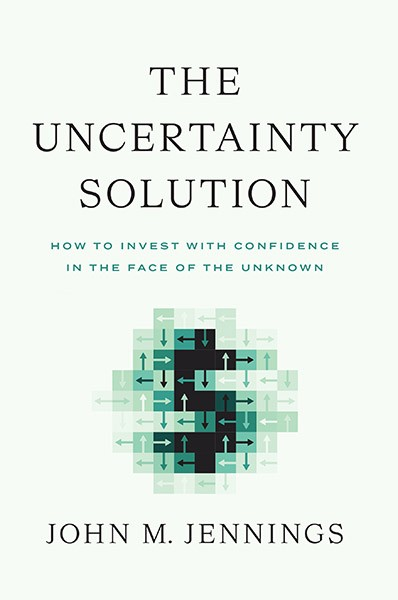## The Goldbach Conjecture

by | Oct 10, 2019

The Goldbach Conjecture is a mathematical concept that seems to be true but remains unproven. It is quite simple – you only need the most rudimentary math skills to understand it.

In 1742 mathematician Christian Goldbach noted in a letter to his fellow mathematician and friend Leonhard Euler that it appeared that all even whole numbers greater than 2 were the sum of two prime numbers.

For example,

• 4 is the sum of 2 and 2 and of course 2 is a prime number. Or, 1 plus 3 gets you there as well and both are primes.
• 18 is the sum of primes 5 and 13 or 7 and 11
• 1,024 is the sum of the primes of 113 and 911

Here’s a fun Goldbach calculator where you can try out whatever even number you want and it will tell you the primes that sum to the number: Goldbach Calculator

So far, a billion billion (1.6 x 1018) whole even numbers have been tested and the Goldbach Conjecture holds for all of them.

But, the Goldbach Conjecture has not been proven true via mathematical formula or logical proof. Until there is a proof there could always be some number out there that fails the rule (which is why its still a conjecture). Famously, Euler’s “sum of powers conjecture” failed at the number 61,917,364,224 (1445). See here for more on that. The paper that was published disproving the conjecture was a mere two sentences long and one of the shortest academic papers in history. Here it is in its entirety:

An example of a beautiful and relatively easy to understand mathematical proof is Euclid’s proof of infinite primes. IFOD on Infinite Primes. The proof is a very beautiful piece of reasoning (a book I read a few years ago said that not knowing of this proof is a gap of experience in your life equivalent to having never tasted chocolate or having heard a single piece of music).

What’s fascinating about Goldbach’s Conjecture and other conclusions based on observation is that you can have millions or billions of observations in favor of the proposed rule but just one observation can kill the rule. A single even number in the trillions of trillions that isn’t the sum of two primes will kill the rule even though there have been a billion billion observations. This is the problem with inductive reasoning. More on the problem of induction here: Turkeys, Swans and the Problem of Induction. In mathematics, to know that something will always be true you must prove that with a proof.

As a side note, in the same letter Goldbach proposed that every odd number greater than 5 can be written as the sum of three prime numbers. In 2013 that version of the Goldbach Conjecture (called the “weak” or “tertiary” Goldbach Conjecture) was proven. Here’s an article on that proof: Click here.

1. Comments on the infinite prime article are closed, but I read it and Euclid’s proof is both simple and brilliant. At least, I didn’t think of it, so he’s smarter than me.

But maybe he thought about it longer than I did.

• Yeah. You and me both. We would have totally come up with that proof.

This site uses Akismet to reduce spam. Learn how your comment data is processed.## View the Archives## Subscribe To The IFOD

Get the Interesting Fact of the Day delivered twice a week. Plus, sign up today and get Chapter 2 of John's book The Uncertainty Solution to not only Think Better, but Live Better. Don't miss a single post!